### Deriving the posterior probabilities of the site types

We will call a variant at a site if the sum of the posterior probabilities of the non-homozygous reference site types is larger than the user-specified cut-off value. For this we need to be able to calculate the posterior site type probabilities. We here derive the formula for these.

Using the Bayesian approach we can write the posterior probability of a site type,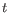, as follows: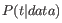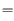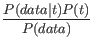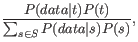(28.1)

where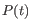is the prior probability of site type(that is,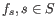, from above) and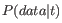is the likelihood of the data, given the site type. The data consists of all the nucleotides in all the reads in the mapping. For a particular site, assume that we have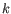reads that cover this site, and let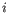be an index over the nucleotides observed,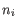, in the reads at this site. We thus have: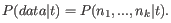To derive the likelihood of the data,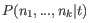, we first need some notation: For a given site type,, let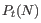be the probability that an allele from this site type has the nucleotide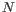. Theprobabilities are known and are determined by the ploidy: For a diploid organism,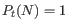ifis a homozygous site andis one of the alleles in, whereas it is 0.5 ifis a heterozygous andis one of the alleles in, and it is 0, ifis not one of the alleles in. For a triploid organism, thewill be either 0, 1/3, 2/3 or 1.

With this definition, we can write the likelihood of the data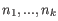in a siteas: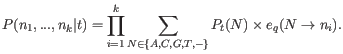(28.2)

Inserting this expression for the likelihood, and the prior site type frequencies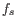and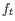for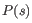and, in the expression for the posterior probability (28.1), we thus have the following equation for the posterior probabilities of the site types: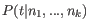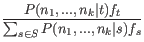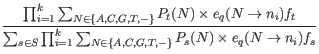(28.3)

The unknowns in this equation are the prior site type probabilities,, and the error rates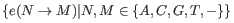. Once these have been estimated, we can calculate the posterior site type probabilities using the equation 28.3 for each site type, and hence, for each site, evaluate whether the sum of the posterior probabilities of the non-homozygous reference site types is larger than the cut-off. If so, we will set out current estimated site type to be that with the highest posterior probability.